## 1. Data description¶

I will analyse California Housing Data (1990). It can be downloaded from Kaggle [https://www.kaggle.com/harrywang/housing]

We will predict the median price of household in block. To start you need to download file housing.csv.zip . Let's load the data and look at it.

In :
import pandas as pd
import numpy as np
import os
%matplotlib inline

import warnings                                  # do not disturbe mode
warnings.filterwarnings('ignore')

In :
# change this if needed
PATH_TO_DATA = 'data'

In :
full_df = pd.read_csv(os.path.join(PATH_TO_DATA, 'housing.csv.zip'), compression ='zip')
print(full_df.shape)

(20640, 10)

Out:
longitude latitude housing_median_age total_rooms total_bedrooms population households median_income median_house_value ocean_proximity
0 -122.23 37.88 41.0 880.0 129.0 322.0 126.0 8.3252 452600.0 NEAR BAY
1 -122.22 37.86 21.0 7099.0 1106.0 2401.0 1138.0 8.3014 358500.0 NEAR BAY
2 -122.24 37.85 52.0 1467.0 190.0 496.0 177.0 7.2574 352100.0 NEAR BAY
3 -122.25 37.85 52.0 1274.0 235.0 558.0 219.0 5.6431 341300.0 NEAR BAY
4 -122.25 37.85 52.0 1627.0 280.0 565.0 259.0 3.8462 342200.0 NEAR BAY

Data consists of 20640 rows and 10 features:

1. longitude: A measure of how far west a house is; a higher value is farther west
2. latitude: A measure of how far north a house is; a higher value is farther north
3. housingMedianAge: Median age of a house within a block; a lower number is a newer building
4. totalRooms: Total number of rooms within a block
5. totalBedrooms: Total number of bedrooms within a block
6. population: Total number of people residing within a block
7. households: Total number of households, a group of people residing within a home unit, for a block
8. medianIncome: Median income for households within a block of houses (measured in tens of thousands of US Dollars)
9. medianHouseValue: Median house value for households within a block (measured in US Dollars)
10. oceanProximity: Location of the house w.r.t ocean/sea

median_house_value is our target feature, we will use other features to predict it.

The task is to predict how much the houses in particular block cost (the median) based on information of blocks location and basic sociodemographic data

Let's divide dataset into train (75%) and test (25%).

In :
%%time
from sklearn.model_selection import train_test_split
train_df, test_df = train_test_split(full_df,shuffle = True, test_size = 0.25, random_state=17)
train_df=train_df.copy()
test_df=test_df.copy()
print(train_df.shape)
print(test_df.shape)

(15480, 10)
(5160, 10)
Wall time: 636 ms


All futher analysis we will do with the test set. But feature generation and processing will be simmultaneously done on both sets.

## 2-3. Primary data analysis / Primary visual data analysis¶

In :
train_df.describe()

Out:
longitude latitude housing_median_age total_rooms total_bedrooms population households median_income median_house_value
count 15480.000000 15480.000000 15480.000000 15480.00000 15330.000000 15480.000000 15480.000000 15480.000000 15480.000000
mean -119.573557 35.636937 28.673256 2637.47177 536.281018 1422.857364 498.022610 3.883162 207066.410724
std 2.007366 2.141362 12.627921 2157.77458 416.009316 1120.156668 378.993113 1.912431 115618.405834
min -124.350000 32.550000 1.000000 2.00000 2.000000 3.000000 2.000000 0.499900 14999.000000
25% -121.810000 33.930000 18.000000 1452.00000 296.000000 786.000000 279.000000 2.567225 119600.000000
50% -118.510000 34.260000 29.000000 2134.00000 434.000000 1164.000000 408.000000 3.543050 179900.000000
75% -118.007500 37.720000 37.000000 3149.25000 647.000000 1725.000000 606.000000 4.766400 265100.000000
max -114.470000 41.950000 52.000000 32054.00000 5419.000000 35682.000000 5050.000000 15.000100 500001.000000
In :
train_df.info()

<class 'pandas.core.frame.DataFrame'>
Int64Index: 15480 entries, 3400 to 10863
Data columns (total 10 columns):
longitude             15480 non-null float64
latitude              15480 non-null float64
housing_median_age    15480 non-null float64
total_rooms           15480 non-null float64
total_bedrooms        15330 non-null float64
population            15480 non-null float64
households            15480 non-null float64
median_income         15480 non-null float64
median_house_value    15480 non-null float64
ocean_proximity       15480 non-null object
dtypes: float64(9), object(1)
memory usage: 1.3+ MB


We can see that most columns has no nan values (except total_bedrooms), most features has float format, only 1 feature is categorical - ocean_proximity.

In :
train_df[pd.isnull(train_df).any(axis=1)].head(10)

Out:
longitude latitude housing_median_age total_rooms total_bedrooms population households median_income median_house_value ocean_proximity
1493 -122.01 37.94 23.0 3741.0 NaN 1339.0 499.0 6.7061 322300.0 NEAR BAY
18177 -122.00 37.36 17.0 2070.0 NaN 797.0 275.0 8.6155 411200.0 <1H OCEAN
20484 -118.72 34.28 17.0 3051.0 NaN 1705.0 495.0 5.7376 218600.0 <1H OCEAN
17840 -121.89 37.44 8.0 2534.0 NaN 1527.0 364.0 7.8532 422800.0 <1H OCEAN
11096 -117.87 33.83 27.0 2287.0 NaN 1140.0 351.0 5.6163 231000.0 <1H OCEAN
18332 -122.16 37.45 47.0 4234.0 NaN 1808.0 1093.0 4.2297 425000.0 NEAR BAY
15975 -122.45 37.77 52.0 2602.0 NaN 1330.0 647.0 3.5435 278600.0 NEAR BAY
4591 -118.28 34.06 42.0 2472.0 NaN 3795.0 1179.0 1.2254 162500.0 <1H OCEAN
12414 -116.21 33.75 22.0 894.0 NaN 830.0 202.0 3.0673 68200.0 INLAND
13311 -117.61 34.08 12.0 4427.0 NaN 2400.0 843.0 4.7147 158700.0 INLAND

There is no obvious reasons for some total_bedrooms to be NaN. The number of NaNs is about 1% of total dataset. Maybe we could just drop this rows or fill it with mean/median values, but let's wait for a while, and deal with blanks after initial data analysis in a smarter manner.

Let's create the list of numeric features names (it will be useful later).

In :
numerical_features=list(train_df.columns)
numerical_features.remove('ocean_proximity')
numerical_features.remove('median_house_value')
print(numerical_features)

['longitude', 'latitude', 'housing_median_age', 'total_rooms', 'total_bedrooms', 'population', 'households', 'median_income']


Let's look at target feature distribition

In :
train_df['median_house_value'].hist()

Out:
<matplotlib.axes._subplots.AxesSubplot at 0x2290d25dc18>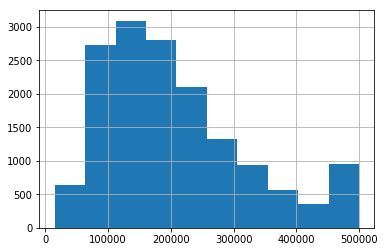We can visually see that distribution is skewed and not normal. Also it seems that the values are clipped somewhere near 500 000. We can check it numerically.

In :
max_target=train_df['median_house_value'].max()
print("The largest median value:",max_target)
print("The # of values, equal to the largest:", sum(train_df['median_house_value']==max_target))
print("The % of values, equal to the largest:", sum(train_df['median_house_value']==max_target)/train_df.shape)

The largest median value: 500001.0
The # of values, equal to the largest: 742
The % of values, equal to the largest: 0.0479328165374677


Almost 5% of all values = exactly 500 001. It proves our clipping theory. Let's check the clipping of small values:

In :
min_target=train_df['median_house_value'].min()
print("The smallest median value:",min_target)
print("The # of values, equal to the smallest:", sum(train_df['median_house_value']==min_target))
print("The % of values, equal to the smallest:", sum(train_df['median_house_value']==min_target)/train_df.shape)

The smallest median value: 14999.0
The # of values, equal to the smallest: 4
The % of values, equal to the smallest: 0.00025839793281653745


This time it looks much better, a little bit artificial value 14 999 - is common for prices. And there are only 4 such values. So probably the small values are not clipped.

Let's conduct some normality tests:

In :
from statsmodels.graphics.gofplots import qqplot
from matplotlib import pyplot

qqplot(train_df['median_house_value'], line='s')
pyplot.show()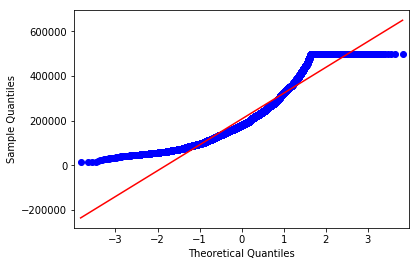In :
from scipy.stats import normaltest

stat, p = normaltest(train_df['median_house_value'])
print('Statistics=%.3f, p=%.3f' % (stat, p))

alpha = 0.05
if p < alpha:  # null hypothesis: x comes from a normal distribution
print("The null hypothesis can be rejected")
else:
print("The null hypothesis cannot be rejected")

Statistics=1831.228, p=0.000
The null hypothesis can be rejected


QQ-plot and D’Agostino and Pearson’s normality test show that the distribution is far from normal. We can try to use log(1+n) to make it more normal:

In :
target_log=np.log1p(train_df['median_house_value'])
qqplot(target_log, line='s')
pyplot.show()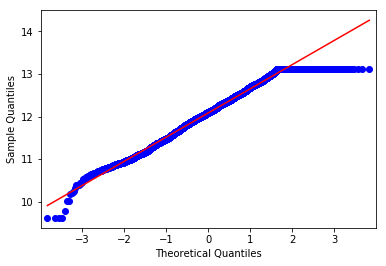In :
stat, p = normaltest(target_log)
print('Statistics=%.3f, p=%.3f' % (stat, p))

alpha = 0.05
if p < alpha:  # null hypothesis: x comes from a normal distribution
print("The null hypothesis can be rejected")
else:
print("The null hypothesis cannot be rejected")

Statistics=252.481, p=0.000
The null hypothesis can be rejected


This graph looks much better, the only non-normal parts are clipped high prices and very low prices. Unfortunately we can not reconstruct clipped data and statistically the distribution it is still not normal - p-value = 0, the null hypothesis of distribution normality can be rejected.

Anyway, predicting of target_log instead of target can be a good choice for us, but we still should check it during model validation phase.

In :
train_df['median_house_value_log']=np.log1p(train_df['median_house_value'])
test_df['median_house_value_log']=np.log1p(test_df['median_house_value'])


Now let's analyze numerical features. First of all we need to look at their distributions.

In :
train_df[numerical_features].hist(bins=50, figsize=(10, 10))

Out:
array([[<matplotlib.axes._subplots.AxesSubplot object at 0x000002290D74A048>,
<matplotlib.axes._subplots.AxesSubplot object at 0x000002290D773940>,
<matplotlib.axes._subplots.AxesSubplot object at 0x000002290D13DFD0>],
[<matplotlib.axes._subplots.AxesSubplot object at 0x000002290D16C6A0>,
<matplotlib.axes._subplots.AxesSubplot object at 0x000002290D194D30>,
<matplotlib.axes._subplots.AxesSubplot object at 0x000002290D194D68>],
[<matplotlib.axes._subplots.AxesSubplot object at 0x000002290D1EEA90>,
<matplotlib.axes._subplots.AxesSubplot object at 0x000002290D21F160>,
<matplotlib.axes._subplots.AxesSubplot object at 0x000002290D7A87F0>]],
dtype=object)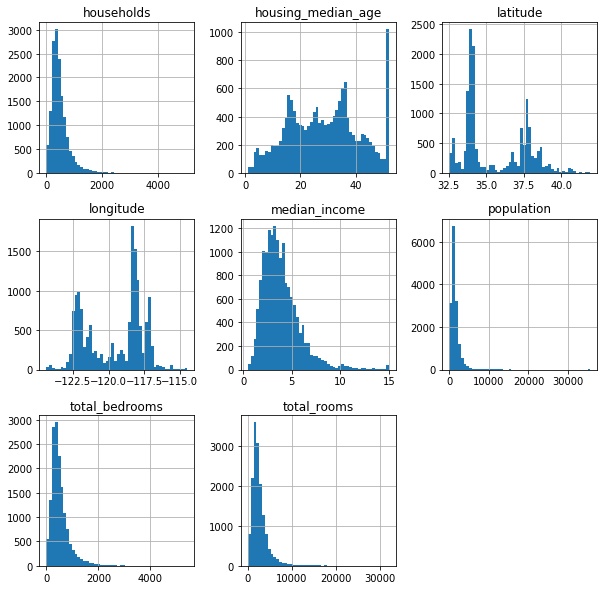Some features are signifacantly skewed, and our "log trick" should be heplfull

In :
skewed_features=['households','median_income','population', 'total_bedrooms', 'total_rooms']
log_numerical_features=[]
for f in skewed_features:
train_df[f + '_log']=np.log1p(train_df[f])
test_df[f + '_log']=np.log1p(test_df[f])
log_numerical_features.append(f + '_log')

In :
train_df[log_numerical_features].hist(bins=50, figsize=(10, 10))

Out:
array([[<matplotlib.axes._subplots.AxesSubplot object at 0x000002290D6CB860>,
<matplotlib.axes._subplots.AxesSubplot object at 0x000002290DB89E10>],
[<matplotlib.axes._subplots.AxesSubplot object at 0x000002290DF86710>,
<matplotlib.axes._subplots.AxesSubplot object at 0x000002290D8E0DA0>],
[<matplotlib.axes._subplots.AxesSubplot object at 0x000002290D907470>,
<matplotlib.axes._subplots.AxesSubplot object at 0x000002290D9074A8>]],
dtype=object)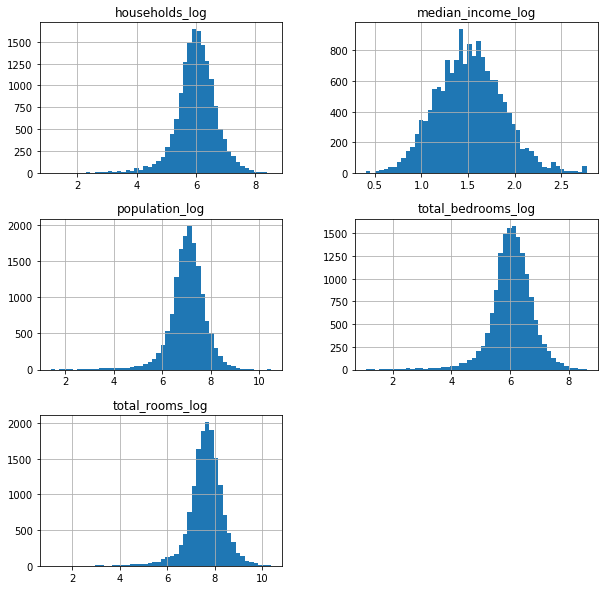Our new features looks much better (during the modeling phase we can use either original, new ones or both of them)

housing_median_age looks clipped as well. Let's look at it's highest value precisely.

In :
max_house_age=train_df['housing_median_age'].max()
print("The largest value:",max_house_age)
print("The # of values, equal to the largest:", sum(train_df['housing_median_age']==max_house_age))
print("The % of values, equal to the largest:", sum(train_df['housing_median_age']==max_house_age)/train_df.shape)

The largest value: 52.0
The # of values, equal to the largest: 978
The % of values, equal to the largest: 0.0631782945736434


It is very likely the data is clipped (there are also a small chance that in 1938 there was a great reconstruction project in California but it seems less likely). We can't recreate original values, but it can be useful to create new binary value indicating the clipping of the house age.

In :
train_df['age_clipped']=train_df['housing_median_age']==max_house_age
test_df['age_clipped']=test_df['housing_median_age']==max_house_age


Now we will analyse correleation between features and target variable

In :
import matplotlib.pyplot as plt
import seaborn as sns

corr_y = pd.DataFrame(train_df).corr()
plt.rcParams['figure.figsize'] = (20, 16)  # Размер картинок
sns.heatmap(corr_y,
xticklabels=corr_y.columns.values,
yticklabels=corr_y.columns.values, annot=True)

Out:
<matplotlib.axes._subplots.AxesSubplot at 0x2290e416438>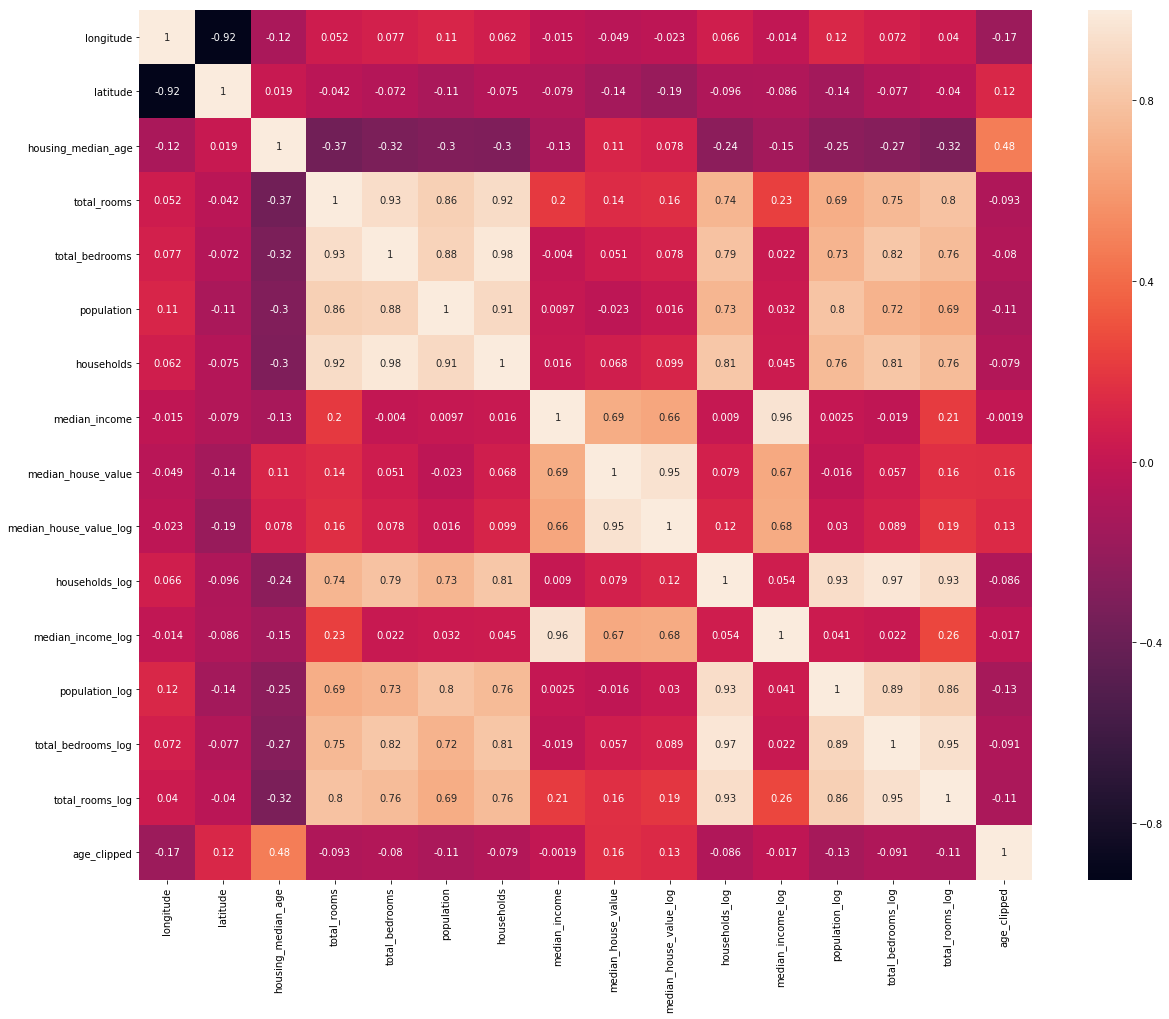We can see some (maybe obvious) patterns here:

- House values are significantly correlated with median income
- Number of households is not 100% correlated with population, we can try to add average_size_of_household as a feature
- Longitude and Latitude should be analyzed separately (just a correlation with target variable is not very useful)
- There is a set of highly correlated features: number of rooms, bedrooms, population and households. It can be useful to reduce dimensionality of this subset, especially if we use linear models
- total_bedrooms is one of these highly correlated features, it means we can fill NaN values with high precision using simplest linear regression

Let's try to fill NaNs with simple linear regression:

In :
from sklearn.linear_model import LinearRegression
from sklearn.metrics import mean_squared_error

lin = LinearRegression()

# we will train our model based on all numerical non-target features with not NaN total_bedrooms
appropriate_columns = train_df.drop(['median_house_value','median_house_value_log',
'ocean_proximity', 'total_bedrooms_log'],axis=1)
train_data=appropriate_columns[~pd.isnull(train_df).any(axis=1)]

# model will be validated on 25% of train dataset
# theoretically we can use even our test_df dataset (as we don't use target) for this task, but we will not
temp_train, temp_valid = train_test_split(train_data,shuffle = True, test_size = 0.25, random_state=17)

lin.fit(temp_train.drop(['total_bedrooms'],axis=1), temp_train['total_bedrooms'])
np.sqrt(mean_squared_error(lin.predict(temp_valid.drop(['total_bedrooms'],axis=1)),
temp_valid['total_bedrooms']))

Out:
64.53336471582273

RMSE on a validation set is 64.5. Let's compare this with the best constant prediction - what if we fill NaNs with mean value:

In :
np.sqrt(mean_squared_error(np.ones(len(temp_valid['total_bedrooms']))*temp_train['total_bedrooms'].mean(),
temp_valid['total_bedrooms']))

Out:
425.3238618340849

Obviously our linear regression approach is much better. Let's train our model on whole train dataset and apply it to the rows with blanks. But preliminary we will "remember" the rows with NaNs, because there is a chance, that it can contain useful information.

In :
lin.fit(train_data.drop(['total_bedrooms'],axis=1), train_data['total_bedrooms'])

train_df['total_bedrooms_is_nan']=pd.isnull(train_df).any(axis=1).astype(int)
test_df['total_bedrooms_is_nan']=pd.isnull(test_df).any(axis=1).astype(int)

train_df['total_bedrooms'].loc[pd.isnull(train_df).any(axis=1)]=\
lin.predict(train_df.drop(['median_house_value','median_house_value_log','total_bedrooms','total_bedrooms_log',
'ocean_proximity','total_bedrooms_is_nan'],axis=1)[pd.isnull(train_df).any(axis=1)])

test_df['total_bedrooms'].loc[pd.isnull(test_df).any(axis=1)]=\
lin.predict(test_df.drop(['median_house_value','median_house_value_log','total_bedrooms','total_bedrooms_log',
'ocean_proximity','total_bedrooms_is_nan'],axis=1)[pd.isnull(test_df).any(axis=1)])

#linear regression can lead to negative predictions, let's change it
test_df['total_bedrooms']=test_df['total_bedrooms'].apply(lambda x: max(x,0))
train_df['total_bedrooms']=train_df['total_bedrooms'].apply(lambda x: max(x,0))


Let's update 'total_bedrooms_log' and check if there are no NaNs left

In :
train_df['total_bedrooms_log']=np.log1p(train_df['total_bedrooms'])
test_df['total_bedrooms_log']=np.log1p(test_df['total_bedrooms'])

In :
print(train_df.info())
print(test_df.info())

<class 'pandas.core.frame.DataFrame'>
Int64Index: 15480 entries, 3400 to 10863
Data columns (total 18 columns):
longitude                 15480 non-null float64
latitude                  15480 non-null float64
housing_median_age        15480 non-null float64
total_rooms               15480 non-null float64
total_bedrooms            15480 non-null float64
population                15480 non-null float64
households                15480 non-null float64
median_income             15480 non-null float64
median_house_value        15480 non-null float64
ocean_proximity           15480 non-null object
median_house_value_log    15480 non-null float64
households_log            15480 non-null float64
median_income_log         15480 non-null float64
population_log            15480 non-null float64
total_bedrooms_log        15480 non-null float64
total_rooms_log           15480 non-null float64
age_clipped               15480 non-null bool
total_bedrooms_is_nan     15480 non-null int32
dtypes: bool(1), float64(15), int32(1), object(1)
memory usage: 2.1+ MB
None
<class 'pandas.core.frame.DataFrame'>
Int64Index: 5160 entries, 18403 to 15058
Data columns (total 18 columns):
longitude                 5160 non-null float64
latitude                  5160 non-null float64
housing_median_age        5160 non-null float64
total_rooms               5160 non-null float64
total_bedrooms            5160 non-null float64
population                5160 non-null float64
households                5160 non-null float64
median_income             5160 non-null float64
median_house_value        5160 non-null float64
ocean_proximity           5160 non-null object
median_house_value_log    5160 non-null float64
households_log            5160 non-null float64
median_income_log         5160 non-null float64
population_log            5160 non-null float64
total_bedrooms_log        5160 non-null float64
total_rooms_log           5160 non-null float64
age_clipped               5160 non-null bool
total_bedrooms_is_nan     5160 non-null int32
dtypes: bool(1), float64(15), int32(1), object(1)
memory usage: 710.5+ KB
None


After filling of blanks let's have a closer look on dependences between some numerical features

In :
sns.set()
sns.pairplot(train_df[log_numerical_features+['median_house_value_log']])

Out:
<seaborn.axisgrid.PairGrid at 0x2290e8f5e48>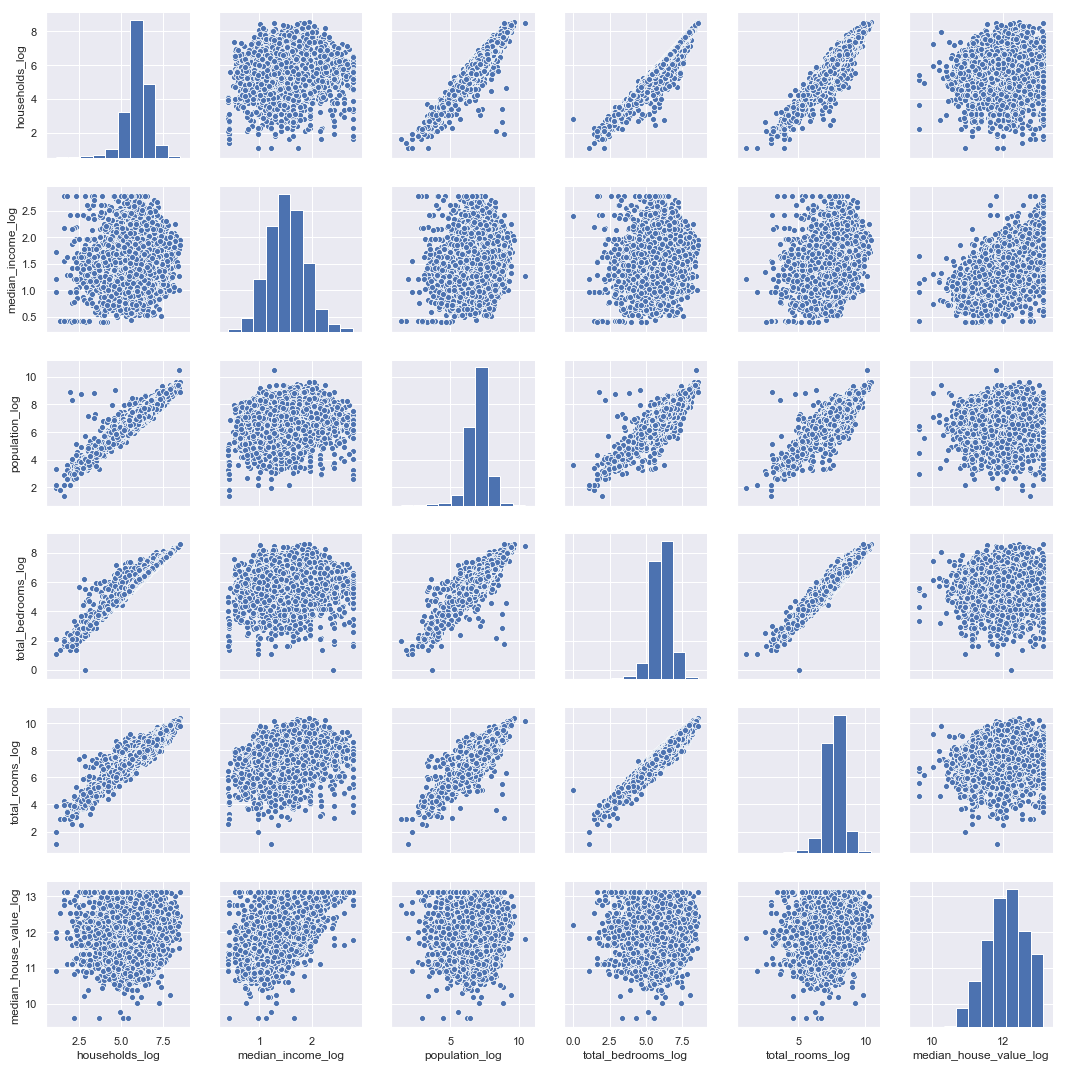It seems there are no new insights about numerical features (only confirmation of the old ones).

Let's try to do the same thing but for the local (geographically) subset of our data.

In :
sns.set()
local_coord=[-122, 41] # the point near which we want to look at our variables
euc_dist_th = 2 # distance treshhold

euclid_distance=train_df[['latitude','longitude']].apply(lambda x:
np.sqrt((x['longitude']-local_coord)**2+
(x['latitude']-local_coord)**2), axis=1)

# indicate wethere the point is within treshhold or not
indicator=pd.Series(euclid_distance<=euc_dist_th, name='indicator')

print("Data points within treshhold:", sum(indicator))

# a small map to visualize th eregion for analysis
sns.lmplot('longitude', 'latitude', data=pd.concat([train_df,indicator], axis=1), hue='indicator', markers ='.', fit_reg=False, height=5)

# pairplot
sns.pairplot(train_df[log_numerical_features+['median_house_value_log']][indicator])

Data points within treshhold: 500

Out:
<seaborn.axisgrid.PairGrid at 0x229123474e0>